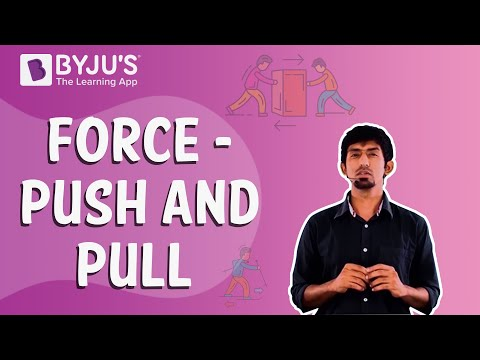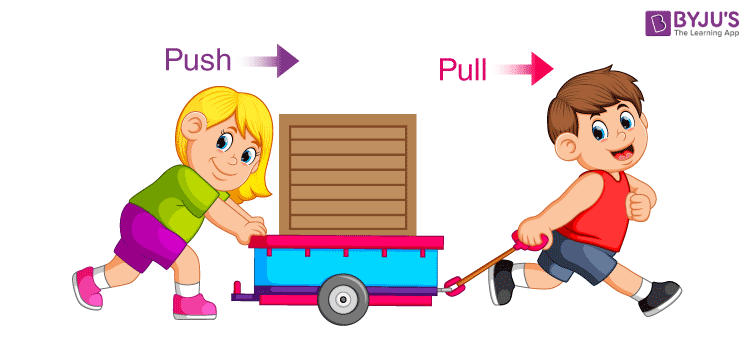# Force : Push and Pull Action

## What is Force?

Force is an important concept as it influences motion. It can be defined as an interaction that changes the motion of an object if unopposed. But the simple definition of force is that it is the push or pull experienced by any object. Force is a vector quantity, thus it has both magnitude and direction. Therefore, one has to specify both the direction and the magnitude to describe the force acting on an object.### Types of Force

By applying force, one can make an object at rest move. This application of force can be done either by touching the object or by without touching the object. Therefore, there are two types of forces:

1. Contact force
2. Non-contact force

#### Contact Force

The force that is applied to the objects by bringing them into contact. There are three types of contact forces:

1.  Frictional force
2. Applied force
3. Normal force

#### Non-Contact Force

The force which is applied to the body without coming in contact is known as non-contact force. Gravitational force is an example of non-contact force.

### Force: Push and Pull

For instance, force has been defined as an interaction that changes the motion of an object if unopposed. When this statement is examined closely, we see the role of push-pull in this. A force that changes the direction of an object towards you, would be a pull. On the other hand, if it moves away, it is a push. Sometimes, force is simply defined as a push or pull upon an object resulting from the object’s interaction with another object. Hence, any kind of force is basically a push or a pull. Spring and elastic are also types of forces. The moment you push against it, it tends to resist and react or spring back with the same magnitude.### Push and Pull Examples

Push is defined as the force that is responsible for an object moving from the state of rest.

#### Examples of push:

• Pushing the trolley.
• Pushing of the car when it breaks down.
• Pushing the table from one place to another.

The pull is defined as the force that is responsible for an object moving from the state of rest but in the opposite direction when compared to the push.

#### Examples of pull:

• Pulling the curtain.
• Dragging the box.
• Opening of the door.

Whenever we consider a force in a given scenario, it can act as an internal as well as an external force depending on the system we have considered. This is how we have introduced this topic from the basics and it emphasizes the fact that we have to be careful about the system chosen whenever we are labelling a force as an internal or an external force.## Frequently Asked Questions – FAQs

Q1

### What is a force?

The push or pull experienced by any object is known as force.
Q2

### Is force a scalar or vector quantity?

Force is a vector quantity since it has both magnitude and direction.
Q3

### What are the types of force?

Types of force are:
• Contact force
• Non-contact force
• Q4

### What are the types of contact forces?

Types of contact forces are:
• Frictional force
• Applied force
• Normal force
• Q5

### What type of force is the gravitational force?

Gravitational force is a non-contact force.

Test Your Knowledge On Force Push And Pull!